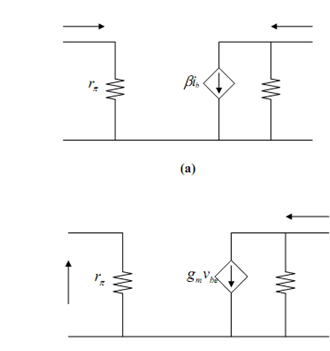## Transconductance Small Signal Model Assignment Help

Assignment Help: >> Small Signal Equivalent Model - Transconductance Small Signal Model

Transconductance Small Signal Model:

Back in the very straining of the transistor era, an engineer at Jim Early, Bell Labs, predicted that there would be a slope to the IC curves, and that they would all of the project back to the same intersection point on the horizontal axis. Having built that prediction, Jim went down into the laboratory, made the measurement, and confirmed his prediction, therefore showing that the theory of transistor behaviour was being correctly understood. The point of intersection of the VCE axis is known as the Early Voltage. As the symbol VE, for the emitter voltage was already taken, they ought to label the Early Voltage VA instead. (Even though the intersection points are on the negative half of the VCE axis, VA is universally quoted as a positive number.)

Generally, the slope is much less than we have illustrated here, and so for any given value of IC, we can just take the slope of the line as IC/VA, and therefore the resistance, which is usually called r0 is just VA/Ic. Therefore, we add r0 to the small signal model for the bipolar transistor. This is illustrated in Figure (a). In a good quality modern transistor, the Early Voltage, VA shall be on the order of 150-250 Volts. So if we let VA = 200 V, and we suppose that we have our transistor biased at 1 mA, then r0 =200 V/1 mA = 200 k?, which is usually much larger than most of the other resistors you shall encounter in a typical circuit. In most instances, r0 can be ignored with no problem.(b)

Figure: (a) Including r0 in the Small Signal Model; and (b) Transconductance Small Signal Model

Sometimes this is advantageous to use a mutual transconductance model of Figure (b) rather than a current gain model for the transistor. If we say the input small signal voltage is v, then obviously

i  = vBE / rπ  =   vBE / (β/ 40IC)

But

i  = βib  = β vbe  / (β /40Ic) =40Ic vbe = gmvbe

where gm is called the mutual transconductance of the transistor. Notice down that β has totally cancelled out in the expression for gm and that gm based only upon the bias current, IC, passing through the collector and not on any of the physical properties of the transistor itself.